# PQ 树

PQ 树是一种基于树的数据结构，代表一组元素上的一系列排列，由 Kellogg S. Booth 和 George S. Lueker 于 1976 年发现命名，用来解决以下问题

PQ 树可以在 $O(n+\sum|S_i|)$ 时间内构建。本文中介绍的构建方法时间复杂度为 $O(nm)$

## 定义¶

PQ 树有三种结点：叶子结点P 结点Q 结点。其中叶子结点代表排列中的一个元素，P 结点表示它的子结点可以任意排列，Q 结点表示它的儿子顺序可以反转。所有非叶子结点都是 P 结点或 Q 结点中的一种。P 结点至少有 2 个儿子，Q 结点至少有 3 个儿子。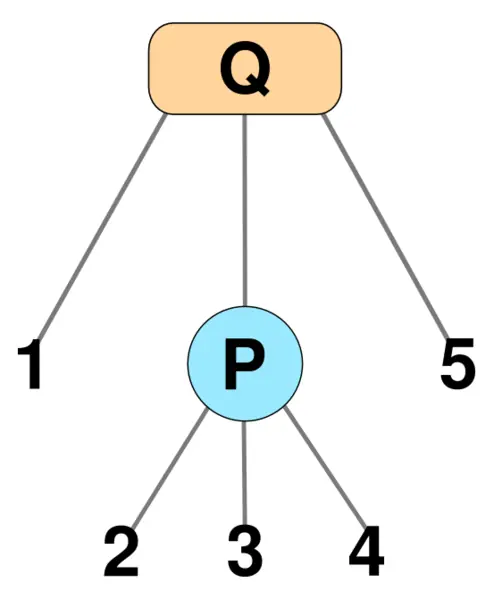## 构建¶

PQ 树使用儿子 - 兄弟表示法。

### 自底向上法¶

#### 减少阶段¶

##### 叶子结点¶

$u$ 标记为黑色。

##### P 结点¶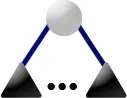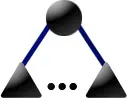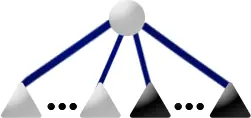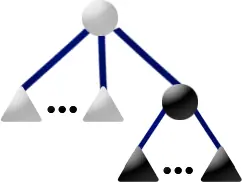• 新建一个 P 结点 $f$ 成为所有黑色儿子的根。
• 新建一个 P 结点 $e$ 成为所有白色儿子的根。
• 如果 $e$（和/或 $f$）只有一个儿子，那么不要新建结点，而是将 $e$（和/或 $f$）直接赋值成那个儿子。
• $u$ 改成 Q 结点并把其儿子设为 $e$$f$，将其标记为灰色。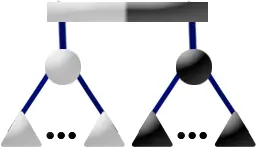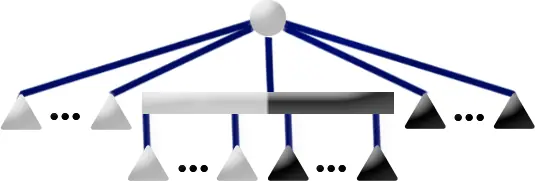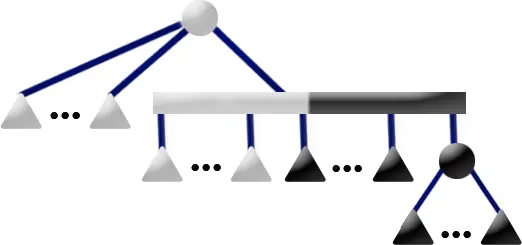• 新建一个 P 结点 $f$ 成为所有黑色儿子的根。
• 新建一个 P 结点 $e$ 成为所有白色儿子的根。
• 如果 $e$（和/或 $f$）只有一个儿子，那么不要新建结点，而是将 $e$（和/或 $f$）直接赋值成那个儿子。
• $e$ 的兄弟设为 $p$ 最后一个白色儿子，然后把 $e$ 设为 $p$ 的最后一个儿子。
• $f$ 的兄弟设为 $p$ 最后一个黑色儿子，然后把 $f$ 设为 $p$ 的最后一个儿子。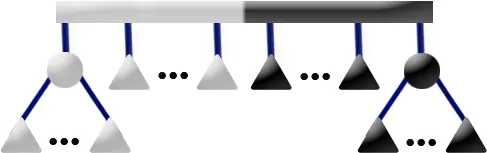• 新建一个 P 结点 $f$ 成为所有黑色儿子的根。
• 如果 $f$ 只有一个儿子，那么不要新建结点，而是将 $f$ 直接赋值成那个儿子。
• $p_1$ 的最后一个黑色儿子的兄弟设为 $f$
• $f$ 的兄弟设为 $p_2$ 的最后一个黑色儿子。
• $p_2$ 的最后一个儿子设为 $p_2$ 的最后一个白色儿子。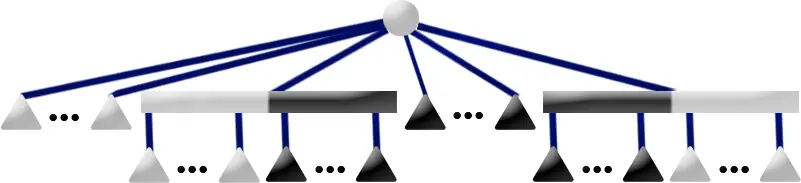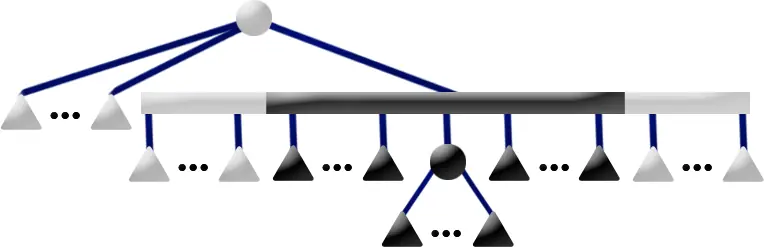##### Q 结点¶• $p_f$$p$ 最后一个黑色儿子，$p_e$$p$ 最后一个白色儿子，$f$$p$ 的黑色兄弟，$e$$p$ 的白色兄弟。
• $f$ 的兄弟设为 $p_f$$e$ 的兄弟设为 $p_e$
• 如果 $p$ 没有一个白色兄弟或黑色兄弟，将 $u$ 的最后一个儿子设成 $p$ 的最后一个儿子。
• 删除 $p$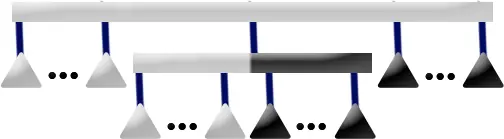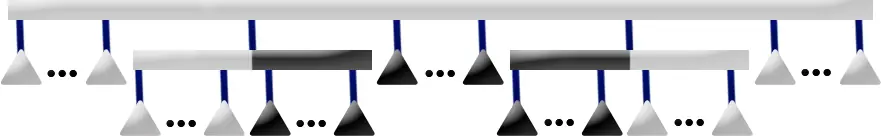### 自顶向下法¶

#### P 结点¶

• 如果 $u$ 有多于两个灰色儿子，无解。
• 如果 $u$ 只有一个灰色儿子，且没有黑色儿子，递归处理灰色儿子。
• 否则先清空 $u$ 的儿子，然后加入所有的白色儿子。新建一个 Q 结点 $q_1$ 并成为 $u$ 的儿子。在 $q_1$ 中加入所有的灰色儿子。新建一个 P 结点 $p$ 作为所有黑色儿子的根，将 $p$ 插入 $q_1$ 的中间。（对应了自底向上法 P 结点的所有情况。）

#### Q 结点¶

• 找到最左边和最右边的非白色节点位置 $l,r$。如果 $[l+1,r-1]$ 内有非黑色节点，无解。
• 如果没有黑色节点，只有一个灰色节点，递归处理这个灰色节点，否则只需要将 $l$$r$ 位置的节点分裂。

#### 分裂函数¶

• 如果 $u$ 有至少两个灰色儿子，则无解。
• 否则左边是所有白色儿子，中间递归处理灰色儿子，右边是所有黑色儿子。注意到要保留所有的可能，因此要新建两个 P 结点分别作为白色儿子和黑色儿子的根。（对应自底向上法的 P4 情况。）
• 删除 $u$

• 如果正序和反序都不满足白 - 灰 - 黑，则无解。
• 如果有至少两个灰色儿子，也无解。
• 否则递归分裂灰色儿子即可。
• 删除 $u$

## 代码实现¶

  1 2 3 4 5 6 7 8 9 10 11 12 13 14 15 16 17 18 19 20 21 22 23 24 25 26 27 28 29 30 31 32 33 34 35 36 37 38 39 40 41 42 43 44 45 46 47 48 49 50 51 52 53 54 55 56 57 58 59 60 61 62 63 64 65 66 67 68 69 70 71 72 73 74 75 76 77 78 79 80 81 82 83 84 85 86 87 88 89 90 91 92 93 94 95 96 97 98 99 100 101 102 103 104 105 106 107 108 109 110 111 112 113 114 115 116 117 118 119 120 121 122 123 124 125 126 127 128 129 130 131 132 133 134 135 136 137 138 139 140 141 142 143 144 145 146 147 148 149 150 151 152 153 154 155 156 157 158 159 160 161 162 163 164 165 166 167 168 169 170 171 172 173 174 175 176 177 178 179 180 181 182 183 184 185 186 187 188 189 190 191 192 193 194 class PQTree { public: PQTree() {} void Init(int n) { n_ = n, rt_ = tot_ = n + 1; for (int i = 1; i <= n; i++) g_[rt_].emplace_back(i); } void Insert(const std::string &s) { s_ = s; Dfs0(rt_); Work(rt_); while (g_[rt_].size() == 1) rt_ = g_[rt_]; Remove(rt_); } std::vector ans() { DfsAns(rt_); return ans_; } ~PQTree() {} private: int n_, rt_, tot_, pool_, top_, typ_ /* 0-P 1-Q */, col_ /* 0-black 1-white 2-grey */; std::vector g_, ans_; std::string s_; void Fail() { std::cout << "NO\n"; std::exit(0); } int NewNode(int ty) { int x = top_ ? pool_[top_--] : ++tot_; typ_[x] = ty; return x; } void Delete(int u) { g_[u].clear(), pool_[++top_] = u; } void Dfs0(int u) { // get color of each node if (u >= 1 && u <= n_) { col_[u] = s_[u] == '1'; return; } bool c0 = false, c1 = false; for (auto &&v : g_[u]) { Dfs0(v); if (col_[v]) c1 = true; if (col_[v] != 1) c0 = true; } if (c0 && !c1) col_[u] = 0; else if (!c0 && c1) col_[u] = 1; else col_[u] = 2; } bool Check(const std::vector &v) { int p2 = -1; for (int i = 0; i < static_cast(v.size()); i++) if (col_[v[i]] == 2) { if (p2 != -1) return false; p2 = i; } if (p2 == -1) for (int i = 0; i < static_cast(v.size()); i++) if (col_[v[i]]) { p2 = i; break; } for (int i = 0; i < p2; i++) if (col_[v[i]]) return false; for (int i = p2 + 1; i < static_cast(v.size()); i++) if (col_[v[i]] != 1) return false; return true; } std::vector Split(int u) { if (col_[u] != 2) return {u}; std::vector ng; if (typ_[u]) { // Q if (!Check(g_[u])) { std::reverse(g_[u].begin(), g_[u].end()); if (!Check(g_[u])) Fail(); } for (auto &&v : g_[u]) if (col_[v] != 2) { ng.emplace_back(v); } else { auto s = Split(v); ng.insert(ng.end(), s.begin(), s.end()); } } else { // P std::vector son; for (auto &&x : g_[u]) son[col_[x]].emplace_back(x); if (son.size() > 1) Fail(); if (!son.empty()) { int n0 = NewNode(0); g_[n0] = son; ng.emplace_back(n0); } if (!son.empty()) { auto s = Split(son); ng.insert(ng.end(), s.begin(), s.end()); } if (!son.empty()) { int n1 = NewNode(0); g_[n1] = son; ng.emplace_back(n1); } } Delete(u); return ng; } void Work(int u) { if (col_[u] != 2) return; if (typ_[u]) { // Q int l = 1e9, r = -1e9; for (int i = 0; i < static_cast(g_[u].size()); i++) if (col_[g_[u][i]]) checkmin(l, i), checkmax(r, i); for (int i = l + 1; i < r; i++) if (col_[g_[u][i]] != 1) Fail(); if (l == r && col_[g_[u][l]] == 2) { Work(g_[u][l]); return; } std::vector ng; for (int i = 0; i < l; i++) ng.emplace_back(g_[u][i]); auto s = Split(g_[u][l]); ng.insert(ng.end(), s.begin(), s.end()); for (int i = l + 1; i < r; i++) ng.emplace_back(g_[u][i]); if (l != r) { s = Split(g_[u][r]); std::reverse(s.begin(), s.end()); ng.insert(ng.end(), s.begin(), s.end()); } for (int i = r + 1; i < static_cast(g_[u].size()); i++) ng.emplace_back(g_[u][i]); g_[u] = ng; } else { // P std::vector son; for (auto &&x : g_[u]) son[col_[x]].emplace_back(x); if (son.empty() && son.size() == 1) { Work(son); return; } g_[u].clear(); if (son.size() > 2) Fail(); g_[u] = son; int n1 = NewNode(1); g_[u].emplace_back(n1); if (son.size() >= 1) { auto s = Split(son); g_[n1].insert(g_[n1].end(), s.begin(), s.end()); } if (son.size()) { int n2 = NewNode(0); g_[n1].emplace_back(n2); g_[n2] = son; } if (son.size() >= 2) { auto s = Split(son); std::reverse(s.begin(), s.end()); g_[n1].insert(g_[n1].end(), s.begin(), s.end()); } } } void Remove(int u) { // remove the nodes with only one child for (auto &&v : g_[u]) { int tv = v; while (g_[tv].size() == 1) { int t = tv; tv = g_[tv]; Delete(t); } v = tv, Remove(v); } } void DfsAns(int u) { if (u >= 1 && u <= n_) { ans_.emplace_back(u); return; } for (auto &&v : g_[u]) DfsAns(v); } } T;